• margin-right:0">请问这是matlab解常微分方程的哪一种方法 ; margin-right:0">clc ; margin-right:0">clear all ; margin-right:0">g=9.8; ; margin-right:0">m=1; ; margin-right:0">C=0.5; ; ...
• ## Matlab解常微分方程

千次阅读 2016-11-07 22:14:02
dsolve('equation','v') 给出微分方程的解析，表示为v的函数 dsolve('equation','condition','v') 例子： 计算微分方程dy/dx+3xy=xexp(-x*x)的通： 计算微分方程x*dy+2y-exp(x)=0在初始
dsolve('equation')
dsolve('equation','condition')
dsolve('equation','v')  给出微分方程的解析解，表示为v的函数
dsolve('equation','condition','v')
例子：
计算微分方程dy/dx+3xy=xexp(-x*x)的通解：

计算微分方程x*dy+2y-exp(x)=0在初始条件y(1)=2e的特解：

>> dsolve('Dy+3*x*y=x*exp(-x^2)','x')

ans =

C2*exp(-(3*x^2)/2) + exp(x^2/2)*exp(-(3*x^2)/2)

>> dsolve('x*Dy+2*y-exp(x)=0','y(1)=2*exp(1)','x')

ans =

(2*exp(1))/x^2 + (exp(x)*(x - 1))/x^2

>> dsolve('D2y+2*Dy+exp(x)=0','x')

ans =

C6 - exp(x)/3 + C7*exp(-2*x)
展开全文• 应用matlab软件对常微分方程求解;前沿;一常微分方程组的符号(4)若边界条件少于方程组的阶数则返回的结果r中会出现任意常数C1C2(6)若没有给定输出参量则在命令窗口显示列表若该命令找不到解析则返回一警告信息...
• 用matlab可以解决许多数学问题，本文章主要讲解利用matlab解常微分方程 1、首先得介绍一下，在matlab中解常微分方程有两种方法，一种是符号解法，另一种是数值解法。在本科阶段的微分数学题，基本上可以通过符号...

用matlab可以解决许多数学问题，本文章主要讲解利用matlab解常微分方程

1、首先得介绍一下，在matlab中解常微分方程有两种方法，一种是符号解法，另一种是数值解法。在本科阶段的微分数学题，基本上可以通过符号解法解决。

2、用matlab解决常微分问题的符号解法的关键命令是dsolve命令。该命令中可以用D表示微分符号，其中D2表示二阶微分，D3表示三阶微分，以此类推。值得注意的是该微分默认是对自变量t求导，也可以很容易在命令中改为对其他变量求导。

3、说了半天，该命令的最完整的形式如下。r=dsolve('eqn1','eqn2',...,'cond1','cond2',...,'var').

解释如下：eqni表示第i个微分方程，condi表示第i个初始条件，var表示微分方程中的自变量，默认为t。

4、解释了半天，不如用实例来说明。下面以解决一个简单的微分方程入手。方程如下。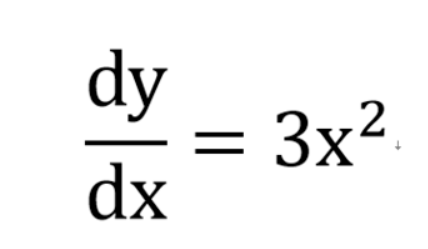5、 首先启动matlab软件，在命令行中欢快地敲入

dsolve（‘Dy=3*x*x’，‘x’）,然后轻松地摁一下键盘上的enter建，最后答案就蹦出来了。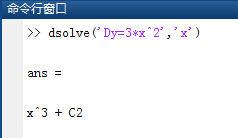6、 下面，我们来加大难度，在上面的方程条件下加入初始条件x=0时，y=2。

只需在相同的命令中加入一个条件语句就可以了。命令形式为

dsolve（‘Dy=3*x*x’，‘y（0）=2’，‘x’）。可以看到答案中的常数项

C2已经变成了2。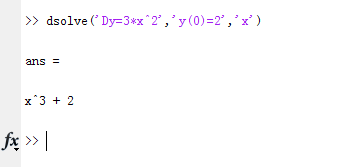7、最后，我再加大一下难度。这次来求一个微分方程组。方程组如下。初始条件为x（0）=2，y（0）=1，dy（0）=1。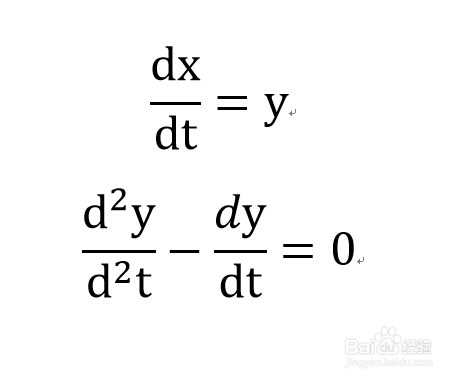8、在命令中只需改一下相应的方程与条件即可以了，由于是对t求导，所以可以用默认，不需要在末尾添加‘t’。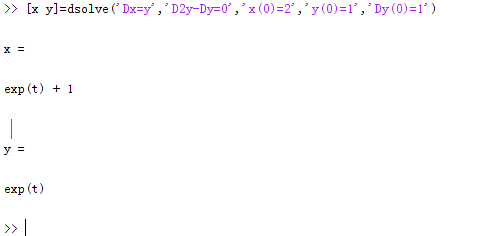展开全文• 1.4.8 解常微分方程 (ordinary differential equation, ODE) 一引言 微分方程求解的数值算法有多种常用的有Euler欧拉法Runge Kutta(龙格-库塔法 Euler法称一步法用于一阶微分方程 0 x0 x1 x2 xn xn+1 当给定步长时 ...
• 微分方程（组）的解析解法 精确 Matlab 有专门的函数可以用 本实验将作一定的介绍． 本实验将主要研究微分方程 组 的数值解法（近似） 重点介绍 Euler 折线法．">我的作业 分享下一 问题背景与实验目的 实际...
• matlab解常微分方程,调用dsolve是老出错 y=dsolve,用matlab解微分方程y=dsolve(’D2y+Dy2用matlab解常微分方程,调用dsolve是老出错 y=dsolve('Dy=x*sin(x)/cos(y...,用matlab解微分方程y=dsolve('D2y+Dy-2y=2x','y...
导航：网站首页 >用matlab解常微分方程,调用dsolve是老出错 y=dsolve,用matlab解微分方程y=dsolve(’D2y+Dy2用matlab解常微分方程,调用dsolve是老出错 y=dsolve('Dy=x*sin(x)/cos(y...,用matlab解微分方程y=dsolve('D2y+Dy-2y=2x','y(0)=0,Dy(0)=1','x')为什...匿名网友:大哥，你是中英文不分吗？你的程序没错，错的是你把里面很多的括号打成了中文dsolve('200000*D2x+4.5*(Dx)^2=0','200000*D2y=-2000000+4.5*(Dy)^2+30*((Dy)^2+(Dx)^2)','Dx(0)=240','x(0)=0','Dy(0)=0','y(0)=10668','t')不过貌似解不了匿名网友:> syms x y a;>> dsolve('Dy=-2*x*y/(x^2+2*a)','x')ans =C1/(x^2+2*a)我这里运行没错，你检查一下你的命令中括号等符号是否有问题，是否有输成全角的情况。另外注意：语句中要加上‘x'，因为matlab默认自变量是t，你这样的式子算出来了也不对。匿名网友:>> syms x y0>> y=dsolve('Dy=y+1/y','y(0)=y0','x')y = (-1+exp(2*x)*(1+y0^2))^(1/2) -(-1+exp(2*x)*(1+y0^2))^(1/2)>> help dsolve DSOLVE Symbolic solution of ordinary differential equations. DSOLVE('eqn1','eqn2', ...) accepts symbolic equations representing ordinary differential equations and initial conditions. Several equations or initial conditions may be grouped together, separated by commas, in a single input argument. By default, the independent variable is 't'. The independent variable may be changed from 't' to some other symbolic variable by including that variable as the last input argument. The letter 'D' denotes differentiation with respect to the independent variable, i.e. usually d/dt. A ＂D＂ followed by a digit denotes repeated differentiation; e.g., D2 is d^2/dt^2. Any characters immediately following these differentiation operators are taken to be the dependent variables; e.g., D3y denotes the third derivative of y(t). Note that the names of symbolic variables should not contain the letter ＂D＂. Initial conditions are specified by equations like 'y(a)=b' or 'Dy(a) = b' where y is one of the dependent variables and a and b are constants. If the number of initial conditions given is less than the number of dependent variables, the resulting solutions will obtain arbitrary constants, C1, C2, etc. Three different types of output are possible. For one equation and one output, the resulting solution is returned, with multiple solutions to a nonlinear equation in a symbolic vector. For several equations and an equal number of outputs, the results are sorted in lexicographic order and assigned to the outputs. For several equations and a single output, a structure containing the solutions is returned. If no closed-form (explicit) solution is found, an implicit solution is attempted. When an implicit solution is returned, a warning is given. If neither an explicit nor implicit solution can be computed, then a warning is given and the empty sym is returned. In some cases involving nonlinear equations, the output will be an equivalent lower order differential equation or an integral. Examples: dsolve('Dx = -a*x') returns ans = C1*exp(-a*t) x = dsolve('Dx = -a*x','x(0) = 1','s') returns x = exp(-a*s) y = dsolve('(Dy)^2 + y^2 = 1','y(0) = 0') returns y =  sin(t)  -sin(t) S = dsolve('Df = f + g','Dg = -f + g','f(0) = 1','g(0) = 2') returns a structure S with fields S.f = exp(t)*cos(t)+2*exp(t)*sin(t) S.g = -exp(t)*sin(t)+2*exp(t)*cos(t) dsolve('Dy = y^2*(1-y^2)') returns Warning:Explicit solution could not be found; implicit solution returned. ans = t+1/2*log(y-1)-1/2*log(y+1)+1/y+C1=0 dsolve('Df = f + sin(t)', 'f(pi/2) = 0') dsolve('D2y = -a^2*y', 'y(0) = 1, Dy(pi/a) = 0') S = dsolve('Dx = y', 'Dy = -x', 'x(0)=0', 'y(0)=1') S = dsolve('Du=v, Dv=w, Dw=-u','u(0)=0, v(0)=0, w(0)=1') w = dsolve('D3w = -w','w(0)=1, Dw(0)=0, D2w(0)=0') y = dsolve('D2y = sin(y)'); pretty(y) See also solve, subs.Reference page in Help browser doc dsolve匿名网友:>>clear>>syms a b c d e;>>y=dsolve('a*D2y+b*Dy+c*y=0','y(0)=d','Dy(0)=e')y =(2*a*e + b*d + d*(b^2 - 4*a*c)^(1/2))/(2*exp((t*(b - (b^2 - 4*a*c)^(1/2)))/(2*a))*(b^2 - 4*a*c)^(1/2)) - (2*a*e + b*d - d*(b^2 - 4*a*c)^(1/2))/(2*exp((t*(b + (b^2 - 4*a*c)^(1/2)))/(2*a))*(b^2 - 4*a*c)^(1/2))>>a=1;b=1;c=1;d=1;e=1；%若常数已知>>t=1;%desolve中没指定自变量x，这里默认为t，带入任意一个x值>>y=eval(y) y =1.1932 + 0.0000i这是解方程的方法，画图也差不多，我在命令窗写的改麻烦，下面是我在editor写的。syms a b c d e;y=dsolve('a*D2y+b*Dy+c*y=0','y(0)=d','Dy(0)=e','x');x=200:0.1:400；%取值范围，步长a=1;b=1;c=1;d=1;e=1；%带入参数y=eval(y);plot(x,y)你补充问题的方程解得也是一个空的，并报错，或许就是无解的，我也很疑问。问题推荐
展开全文• 在数学建模中我遇到了两个数学学习中常见的两个问题，即解常微分方程与数值积分。下面我们来看这两个问题怎么在matlab中得以解决。 除此以外，数学建模中也经常遇到聚类分析，这里也举一个简单的例子。 1.使用ode...
  在数学建模中我遇到了两个数学学习中常见的两个问题，即解常微分方程与数值积分。下面我们来看这两个问题怎么在matlab中得以解决。

除此以外，数学建模中也经常遇到聚类分析，这里也举一个简单的例子。

1.使用ode45()解常微分方程的数值解

【例】解微分方程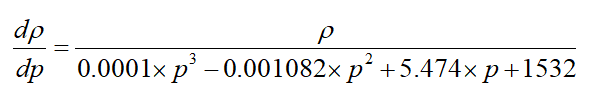初值条件为p(70)=0.8384

【解】

druo=@(p,ruo)(ruo/(0.0001*p^3-0.001082*p^2+5.474*p+1532));
[p,ruo]=ode45(druo,[70 200],0.8384);
plot(p,ruo)
%%插值
p1=70:0.1:200;
ruo1=interp1(p,ruo,p1,'spline');

代码中的前两行是在使用ode45求解微分方程的数值解，没有什么特别好说明的，需要注意，习惯上。在“@（p,ruo）”这一部分中，自变量放在前，因变量放在后。

其中，第一行使用函数句柄定义了需要求解的方程，特别要注意的是调用格式[p,ruo]=ode45(druo,[70 200],0.8384);，在左边的大括号中，自变量放在前，因变量放在后，右边的部分，应该是ode45(函数句柄，[a b],c);其中a和c是对应的，a是初值条件中自变量的值，b是自己指定的任意区间右值，c是初值条件中因变量的值，在这里，由题目给出的初值条件p(70)=0.8384，可知，a=70，c=0.8384，b是自己随便指定的。

2.使用int()求解数值积分

直接给出代码

low=0;  up=5;%积分变量t的上下限

syms t;
p1 =    4.49e-13  ;
p2 =  -7.327e-11 ;
p3 =   9.172e-09  ;
p4 =  -6.245e-07 ;
p5 =  -0.0001539  ;p6 =     -0.1117  ;
p7 =       15.32  ;
Q = p1*t^6 + p2*t^5 + p3*t^4 + p4*t^3 + p5*t^2 + p6*t+ p7;

p1 =  -1.478e-09  ;
p2 =  -1.191e-06 ;
p3 =   0.0003362 ;
p4 =        0.85  ;
rho=p1*t^3+p2*t^2+p3*t+p4;

answer=int(rho*Q,t,low,up);
floor(answer)

【分析】

注意看代码内容，需要用syms t定义符号变量t，然后后面由大量内容只不过是定义了两个函数Q与rho。

事实上的积分，只需要一行代码

answer=int(rho*Q,t,low,up);

int（）的调用格式为int(符号函数,符号自变量,积分下限，积分上限)；

3.kmeans聚类分析

所谓聚类分析，就是把图像上离散的点分为离散的几类，其中内部的原理包含最小二乘法等内容，对于本科生来说没有必要了解其中的细节。

在使用时调用matlab中自带的kmeans函数即可，具体调用格式并不复杂，可以help kmeans查看

展开全文• 在数学建模大赛中，常微分方程的求解至关重要。以下是作者在学习过程中，以SARS病毒传染病SIR模型为例进行的数据处理以及模型求解的过程。算法
• 最近同学毕设需要求解循坏摆的微分方程，我在帮忙过程中学习了一下常微分方程的解析和数值的求法，在此分享。以下讲解遵循Matlab官方文档提供的方程和写法。(强烈建议大家有问题多看官方文档，非常有用)介绍一下...
• 1求解常微分方程MATLAB程序为 2求解常微分方程MATLAB程序为 3求常微分方程组通MATLAB程序为 4求常微分方程组通MATLAB程序为 5求解常微分方程MATLAB程序如下 6求解常微分方程并画出的图形 ?...
• 利用 MATLAB 求解常微分方程数值 目录 1. 内容简介 把高等工程数学看了一遍增加对数学内容的了解对其中数值解法比较感兴趣 这大概是因为在其它各方面的学习和研究中经常会遇到数值解法的问题理解模型然后列出 微分...
• Matlab常微分方程求解中的应用;实验目的 1学会用Matlab软件求解微分方程的初值问题 2了解微分方程数值思想掌握基本的微分方程数值方法 3学会根据实际问题建立简单微分方程数学模型 4了解计算机数据仿真数据...
• PAGE / NUMPAGES Matlab解常微分方程的ode45分析 该文章讲述了Matlab解常微分方程的ode45分析. ode是专门用于解微分方程的功能函数他有ode23,ode45,ode23s等等采用的是Runge-Kutta算法ode45表示采用四阶五阶...
• ## Matlab求常微分方程组的数值解

万次阅读 多人点赞 2020-03-08 09:50:50
上篇博客介绍了Matlab求解常微分方程组解析的方法：博客地址 微分方程组复杂时，无法求出解析时，就需要求其数值，这里来介绍。 以下内容按照Matlab官方文档提供的方程来展开（提议多看官方文档） 介绍一下...经验分享 编程语言
• Matlab常微分方程的解析 最近同学毕设需要求解循坏摆的微分方程，我在帮忙过程中学习了一下常微分方程的解析和数值的求法，在此分享。 以下讲解遵循Matlab官方文档提供的方程和写法。 一阶常微分方程求解...算法
• 本文主要介绍matlab中求解常微分方程（组）的dsolve和ode系列函数，并通过例子加深读者的理解。 一、符号介绍  D: 微分符号；D2表示二阶微分，D3表示三阶微分，以此类推。 二、函数功能介绍及例程 1、dsolve ...
• 常微分方程组数值求解;主要内容 数值求解常微分方程组函数概述 非刚性/刚性常微分方程问题求解 隐式微分方程组求解 微分代数方程(DAE)与延迟微分方程(DDE)求解 边值问题求解 ;第一节数值求解常微分方程组函数概述;一...
• 本文是自己写的关于怎样利用MATLAB求解常微分方程数值的，文中从Euler法讲起，最后总结了常用的odeXX的用法及其原理，其中包含各个函数怎样使用的MATLAB代码
• 数值分析：用Matlab解常微分方程。 该项目是在2015-2016年的一门大学课程（数值方法）中开发的。 注释以西班牙语显示，但的注释以英语显示。 ＃＃职能 funccorazon.m-心形 funcvanderpol.m-范德波尔振荡器 ...
• 首先得介绍一下，在matlab解常微分方程有两种方法，一种是符号解法，另一种是数值解法。在本科阶段的微分数学题，基本上可以通过符号解法解决。 用matlab解决常微分问题的符号解法的关键命令是dslove...
• 练习。使用四阶龙格库塔法求解常微分方程组，通用性较佳。附加一个振动方程求解的案例。振动方程是一个二阶微分方程，转化为两个方程组以后用编写的代码求解。
• 求解如下常微分方程组，其中有s和i两个变量 首先声明这个函数文件 function y=infect(t,x) lamp=11; u=3; y=[lamp*x(1)*x(2)-u*x(1),-lamp*x(1)*x(2)]'; x(1)代表i x(2)代表s 利用ode45对其求数值 ode...函数
• 常微分方程是描述动态系统的常用数学工具, 是很多科学与工程领域数学建模的基础....分方程和低阶特殊常微分方程可以通过解析的方 法求解, 但是一般的非线性常微分方程是没有解析 的, 故需要用数值的方式求解.
• 文章目录前言1 常微分方程1.1 常微分方程的概念1.2 常微分方程数值求解的一般概念2 常微分方程数值求解函数3 刚性...这些近似值称为常微分方程初值问题的数值。相邻两个结点之间的距离称为步长。 口单步法：在计算ya
• 本文主要对简单的连续系统微分方程（含系数矩阵）的MATLAB数值求法进行归纳，并绘制仿真图像。同时也对一阶和高阶微分方程求法进行总结。 系统方程为一阶积分器的连续系统 目录1. 可求解析微分方程2. MATLAB...ieee论文 矩阵
• 微分方程数值第一次报告徐松松41345053计1304 一：实验目的掌握MATLAB语言、C/C++语言编写计算程序的方法、掌握改进欧拉法与四阶龙格-库塔求解一阶常微分方程的初值问题。掌握使用MATLAB程序求解常微分方程问题的...
• 四阶Runge-Kutta法解常微分方程matlab...

# matlab解常微分方程matlab 订阅